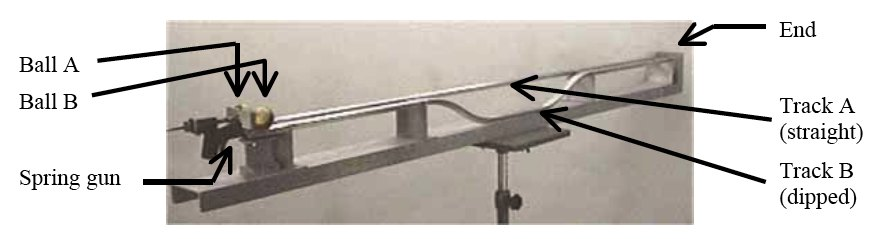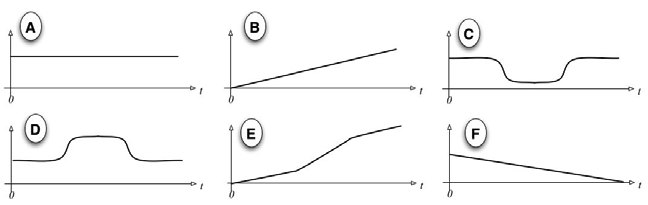Teaching Physics with the Physics Suite Edward F. Redish Problems Sorted by Type | Problems Sorted by Subject | Problems Sorted by Chapter in UP

The dipped track

In a classic lecture demonstration (see C2-11 at the University of Maryland demo site) two billiard balls are driven down parallel tracks by a spring gun. One ball travels along a flat track, the other along a track with a dip. The apparatus is shown in the figure below.Below are shown 6 graphs and 10 physical quantities. Identify which of the graphs could match each physical quantity if the vertical axis were given the correct scale. The time t = 0 occurs just after the balls have been pushed by the spring. Choose the graphs that match each quantity a.-j. If none works, write N. Each graph may be chosen as many times as you like. You may assume friction can be neglected.
 a. The kinetic energy of ball A f. The total energy of ball B b. The potential energy of ball A g. The velocity of ball A c. The kinetic energy of ball B h. The velocity of ball B d. The potential energy of ball B i. The position of ball A e. The total energy of ball A j. The position of ball B.